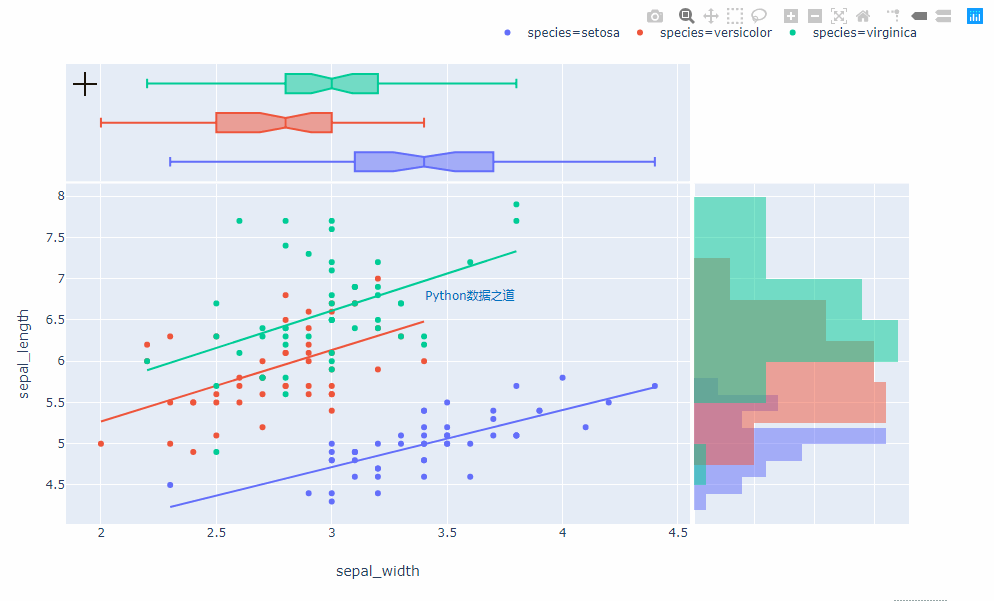# 在 Pycharm 等编辑器下使用 Python 可视化神器 Plotly Express

Plotly

## 第一个示例

# Code based on Python 3.x
# _*_ coding: utf-8 _*_
# Author: "阳哥"
# Website: http://liyangbit.com
# 公众号: Python数据之道
# ID: PyDataLab

import plotly_express as px
import plotly
import plotly.graph_objs as go
plotly.offline.init_notebook_mode(connected=True)

iris = px.data.iris()

iris_plot = px.scatter(iris, x='sepal_width', y='sepal_length',
color='species', marginal_y='histogram',
marginal_x='box', trendline='ols')

plotly.offline.plot(iris_plot)## 第二个示例

# Code based on Python 3.x
# _*_ coding: utf-8 _*_
# Author: "阳哥"
# Website: http://liyangbit.com
# 公众号: Python数据之道
# ID: PyDataLab

import plotly_express as px
import plotly
plotly.offline.init_notebook_mode(connected=True)

wind = px.data.wind()
wind_plot = px.bar_polar(wind, r="value", theta="direction", color="strength", template="plotly_dark",
color_discrete_sequence= px.colors.sequential.Plotly[-2::-1])

plotly.offline.plot(wind_plot)